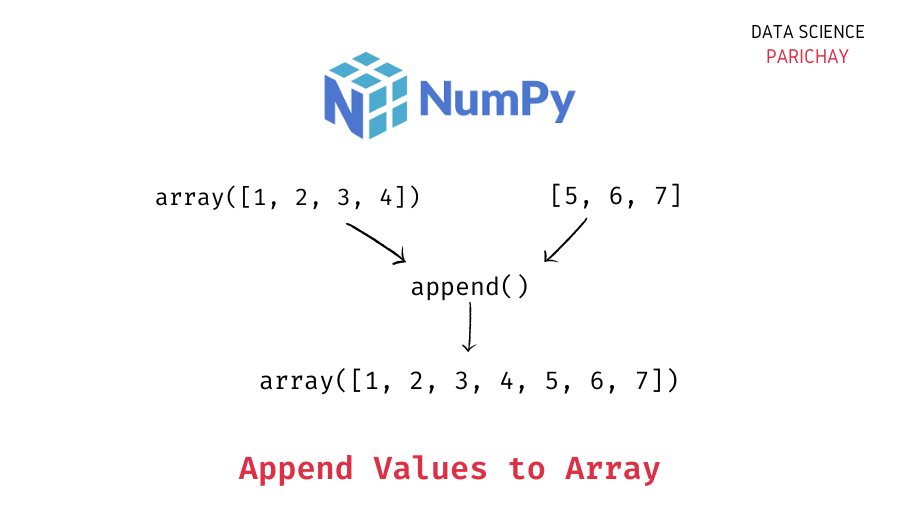# Append Values to a Numpy Array

You can use the numpy `append()` function to append values to a numpy array. In this tutorial, we’ll look at the syntax and usage of the numpy `append()` function through some examples.

It is used to append values at the end of an array. Note that it does not modify the original array. Rather, the values are appended to a copy of the original array and the resulting array is returned. The following is its syntax:

`new_arr = numpy.append(arr, values, axis=None)`

Parameters:

• arr: The original array to append the values on (Values are appended to a copy of this array).
• values: The values to be appended.
• axis (optional): The axis along which the values are to be appended. If the axis is not provided, `arr` and `values` are flattened before appending.

Returns:

A copy of the original array (a numpy ndarray object) with the values appended along the given axis.

Let’s look at some of the use-cases of the `append()` function through examples –

```import numpy as np
# create a sample array
arr = np.array([1,2,3,4])
# append values to arr
new_arr = np.append(arr, [5,6,7])
# print
print("Appended Array:", new_arr)
print("Type:", type(new_arr))```

Output:

📚 Data Science Programs By Skill Level

Introductory

Intermediate ⭐⭐⭐

🔎 Find Data Science Programs 👨‍💻 111,889 already enrolled

Disclaimer: Data Science Parichay is reader supported. When you purchase a course through a link on this site, we may earn a small commission at no additional cost to you. Earned commissions help support this website and its team of writers.

```Appended Array: [1 2 3 4 5 6 7]
Type: <class 'numpy.ndarray'>```

In the above example, note that we didn’t provide an axis. The `append()` function thus flattened the array and value to be appended and returned the resulting array.

Suppose you have a 2D array, and you want to append a new row to it. You can do so by using the `append()` function and setting the `axis` parameter to `0`.

```import numpy as np
# create a sample array
arr = np.array([[1,2,3],[4,5,6]])
# append values to arr
new_arr = np.append(arr, [7,8,9], axis=0)
# print
print("Original array:\n", arr)
print("Appended Array:\n", new_arr)```

Output:

```---------------------------------------------------------------------------
ValueError                                Traceback (most recent call last)
<ipython-input-14-2f4ac95a52b4> in <module>
3 arr = np.array([[1,2,3],[4,5,6]])
4 # append values to arr
----> 5 new_arr = np.append(arr, [7,8,9], axis=0)
6 # print
7 print("Original array:\n", arr)

ValueError: all the input arrays must have same number of dimensions, but the array at index 0 has 2 dimension(s) and the array at index 1 has 1 dimension(s)```

*Some lines in the above error message are skipped to focus on main reason for the error.

When appending values along a specific axis, the array and the values to be appended should have the correct shapes. In the above example, we want to add a row to an array of shape `(2, 3)`, thus, the values to be appended should also have similar dimensions, but the array `[7,8,9]` has shape `(3,)`. To append it as a row, it must be modified so that the dimensions match. See the corrected example below:

```import numpy as np
# create a sample array
arr = np.array([[1,2,3],[4,5,6]])
# append values to arr
new_arr = np.append(arr, [[7,8,9]], axis=0)
# print
print("Original array:\n", arr)
print("Appended Array:\n", new_arr)```

Output:

```Original array:
[[1 2 3]
[4 5 6]]
Appended Array:
[[1 2 3]
[4 5 6]
[7 8 9]]```

Here, the array `[[7,8,9]]` has shape `(1, 3)` which is compatible to be appended as a row to the original `(2, 3)` array.

Suppose you have a 2D array, and you want to append a new column to it. You can do so by using the `append()` function and setting the `axis` parameter to `1`. Like the above example, make sure that the shapes are compatible to be appended along the particular axis.

```import numpy as np
# create a sample array
arr = np.array([[1,1,1],[2,2,2]])
values = np.array([1,2]).reshape(2,1)
# append values to arr
new_arr = np.append(arr, values, axis=1)
# print
print("Original array:\n", arr)
print("Appended Array:\n", new_arr)```

Output:

```Original array:
[[1 1 1]
[2 2 2]]
Appended Array:
[[1 1 1 1]
[2 2 2 2]]```

In the above examples, `values` is reshaped so that it can be appended to the `arr` along `axis=1`.

You know that numpy arrays are objects of the numpy ndarray class but it’s important to keep in mind that `append()` is a function of the numpy class and not the numpy ndarray class. Thus, you cannot directly call the `append()` function from a numpy array like this –

```import numpy as np
arr = np.array([1,2,3,4])
# This gives error
print(arr.append([5,6,7]))```

Output:

```---------------------------------------------------------------------------
AttributeError                            Traceback (most recent call last)
<ipython-input-26-bf9bf23e0201> in <module>
2 arr = np.array([1,2,3,4])
3 # This gives error
----> 4 print(arr.append([5,6,7]))

AttributeError: 'numpy.ndarray' object has no attribute 'append'```

If you want to use the `append()` function, you’ll have call it through numpy directly. Like this –

```import numpy as np
arr = np.array([1,2,3,4])
# This gives error
print(np.append(arr, [5,6,7]))```

Output:

`[1 2 3 4 5 6 7]`

For more on the numpy `append()` function, refer to its official documentation.

With this, we come to the end of this tutorial. The code examples and results presented in this tutorial have been implemented in a Jupyter Notebook with a python (version 3.8.3) kernel having numpy version 1.18.5

•